write an equation of the circle with center (-2,-9) and radius 10

Question

write an equation of the circle with center (-2,-9) and radius 10

in progress 0
6 months 2021-07-15T19:39:29+00:00 2 Answers 2 views 0

Ok Im 95% sure that this is the equation below:

Step-by-step explanation:

(x+2)^2+(y+9)^2=100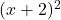+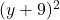= 100

Step-by-step explanation:

Standard Equation of a Circle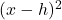+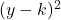=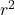(h,k) = center of circle